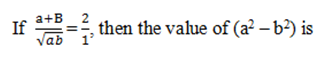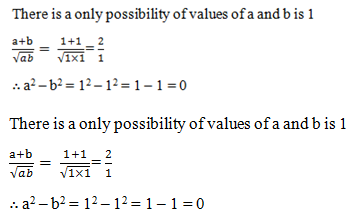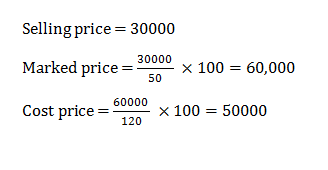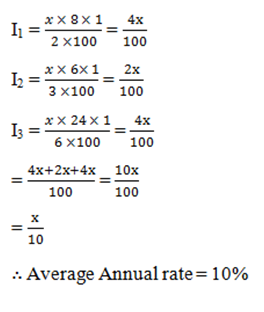# SSC CGL 2018 Practice Test Papers | Quantitative Aptitude (Day-64)

Dear Aspirants, Here we have given the Important SSC CGL Exam 2018 Practice Test Papers. Candidates those who are preparing for SSC CGL 2018 can practice these questions to get more confidence to Crack SSC CGL 2018 Examination.

[WpProQuiz 3832]

Click “Start Quiz” to attend these Questions and view Explanation

1)a) 1

b) 2

c) 3

d) 0

2) If ABC is an equilateral triangle of altitude is 83cm, then what is the area of triangle?

a) 64 √3 cm2

b) 62 √3 cm2

c) 64 √2 cm2

d) 36 √3 cm2

3) Given that tan (θ + 3) = √3. Then the value of θ is ?

a) 45°

b) 30°

c) 60°

d) 15°

4) A Man buys a home theater at the time of stock clearance sale of 50% discount which the selling price is 30000 which is rated as 20% high on original price by the showroom. What is the marked price of the home theater?

a) 45000

b) 30000

c) 50000

d) 75000

5) A tap drips at a rate of one drop/min. 800 drops make 200 ml. What is number of liters wasted in 150 days?

a) 54 litre

b) 14 litre

c) 16 litre

d) 29 litre

6) If 80% of (P+Q) = 120% of (P-Q), then P:Q is equal to

a) 1:5

b) 5:1

c) 2:3

d) 3:2

7) The greatest number that divides 130 and 327 leaving 4 and 3 as remainder respectively, is?

a) 12

b) 18

c) 240

d) 120

8) A man invests half his capital at the rate of 8% per annum. One third at 6% and the rest at 24% per annum. The average rate of interest per annum which he gets is ?

a) 20%

b) 5%

c) 30%

d) 10%

9) The ratio of income of P and Q is 6:5 and the ratio of their expenditure is 3:2. If both of them save Rs. 9000. The expenditure of Q is?

a) 15000

b) 6000

c) 9000

d) 12000

10) The product of two numbers is 135. The sum of their squares is 306. The difference of these two numbers is?

a) 6

b) 12

c) 36

d) 171Length of the altitude of equilateral triangle =  × Side

8 √3 =  × Side

Side = 16 cm

Area of the equilateral triangle =  × a2

Area of the equilateral triangle =  × 16 × 16

Area of the equilateral triangle = 64 √3 cm2

tan (θ + 30°) = √3

tan 60° = √3

θ + 30° = 60°

θ = 30°150 days = 150 × 24 hrs

= 150 × 24 × 60 mins

Number of drops = 150 × 24 × 60

(Since 800 drops = 200 ml

∴ 4 drops = 1 ml)

= (150 × 24 × 60)/4(drops)

= 54000/1000 liters

= 54 liters

80/100(P+Q) = 120/100(P – Q)

80(P+Q) = 120(P – Q)

2(P+Q) = 3(P – Q)

2P + 2Q = 3P – 3Q

5Q = P

∴ P:Q = 5:1

The greatest number that divides means a common divisor HCF. By finding HCF we get the answer 18.Income = x

Expenditure = y

6x – 3y = 9000

5x – 2y = 9000

6x – 3y = 5x – 2y

x = y

3x = 9000  x = 3000

Expenditure of Q, 2x = 2  3000 = 6000

Let the numbers be x and y.

xy = 135

x2 + y2 = 306

(x – y)2 = X2  + y2  – 2xy = 306 – 270

(x – y)2 = 36

x – y = 6